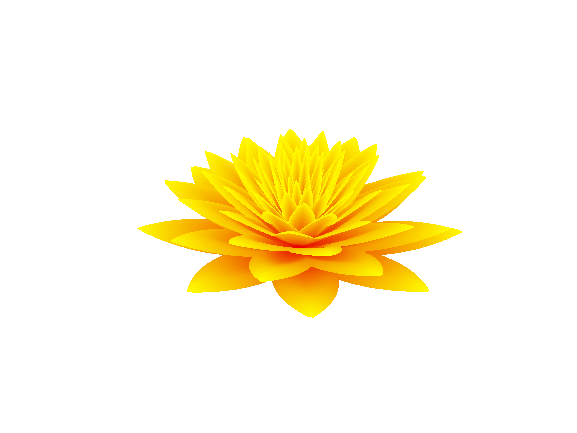# Water LillyEric Ludlam on 27 Oct 2021
• 16
• 123
• 2
• 0
• 273
% Water Lilly
%
% Permutation of the rose (see remix tree) where instead of rounded
% petals, use a simple linear ramp for pointy petals.
% This colonop will be used again for the colormap
m=0:.01:1;
[R,T]=ndgrid(m,0:.01:20);
x=1-abs(1-mod(7.6*T,2))/2;
P=pi*exp(-T/8)/2;
s=@sin;
c=@cos;
u=s(P);
v=c(P);
y=R.^2.*(1.3*R-1).^2.*u;
g=@(f)x.*(R.*u+y.*v).*f(T*pi);
X=g(s);
Y=g(c);
Z=R.*v-y.*u;
surf(X,Y,x.*Z*.6,hypot(hypot(X,Y),Z));
axis equal off
% Create a red to yellow colormap.
%
% This bit of trickery recycles 'm' from above which happened to
% be the same colonop needed for the colormap.
% By adding the special red-ramp into row 3, we auto-fill row 2
% with 0 using no code. Unfortunately that leaves M as a
% purple/blue colormap. Re-arraynge on the way into the colormap
% command to be yellow like some water lillies.
m(3,:)=m*.1+.9;
colormap(m([3
1
2],:)')